search
Search
Guest 0reps
Thanks for the thanks!
close
account_circle
Profile
exit_to_app
Sign out
search
keyboard_voice
close
Searching Tips
Search for a recipe:
"Creating a table in MySQL"
Search for an API documentation: "@append"
Search for code: "!dataframe"
Apply a tag filter: "#python"
Useful Shortcuts
/ to open search panel
Esc to close search panel
to navigate between search results
d to clear all current filters
Enter to expand content previewDoc SearchCode Search BetaSORRY NOTHING FOUND!
mic
Start speaking...Voice search is only supported in Safari and Chrome.
Shrink
Navigate to
A
A
brightness_medium
share
arrow_backShareTwitterFacebook
1
thumb_down
0
chat_bubble_outline
0
auto_stories new
settings

# Plotting in Pandas

Programming
chevron_right
Python
chevron_right
Pandas
chevron_right
Common questions
schedule Jul 4, 2022
Last updated
local_offer PythonPandas
Tags

We can use the `Series.plot(~)` and `DataFrame.plot(~)` methods to easily create plots in Pandas. The `plot(~)` method is a wrapper that allows us to conveniently leverage Matplotlib's powerful charting capabilities.

# Difference between plotting in Pandas and Matplotlib

In short, plotting in Pandas using the `plot(~)` wrapper provides the ability to create plots very easily with a certain degree of customizability. However, if further customization is required, then this will need to be performed directly using the Matplotlib library.

# Dataset

We will be using the classic Iris dataset for the graphs produced throughout this article. It is a commonly used dataset in the machine learning field that contains information on three species of Iris flowers: Setosa, Versicolor, and Virginica.

We load the dataset in as a DataFrame using the below:

``` import numpy as npimport pandas as pddf = pd.read_csv('https://raw.githubusercontent.com/SkyTowner/sample_data/main/iris_dataset')df.head() sepal length (cm) sepal width (cm) petal length (cm) petal width (cm) target0 5.1 3.5 1.4 0.2 setosa1 4.9 3.0 1.4 0.2 setosa2 4.7 3.2 1.3 0.2 setosa3 4.6 3.1 1.5 0.2 setosa4 5.0 3.6 1.4 0.2 setosa ```

# Plot Types

## Line Chart

To create a line chart:

``` ax = df.plot(kind='line', title='Sepal and Petal length / width by record')ax.set_xlabel('Record #') # Add x-axis label ax.set_ylabel('length / width (cm)') # Add y-axis label ```

This produces a line chart like so: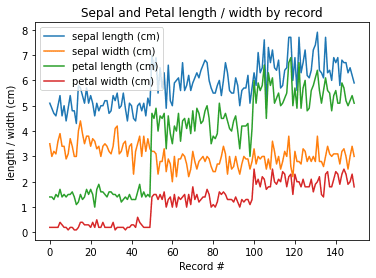NOTE

The default for `df.plot(~)` is to plot a line chart, so removing `kind='line'` from our code would produce exactly the same result.

## Bar Chart

Bar charts are effective when plotting values represented by discrete categories. The bars can be vertical or horizontal.

### Vertical

To create a vertical bar chart use `df.plot(kind='bar')`:

``` ax = df[:5].plot(kind='bar', title='Vertical Bar Chart')ax.set_xlabel('Record #') # Add x-axis label ax.set_ylabel('length / width (cm)') # Add y-axis label ```

This produces the following: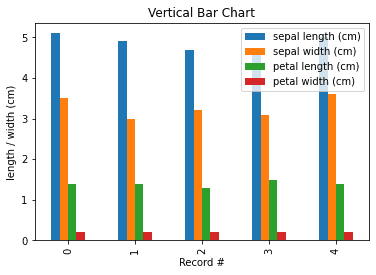NOTE

You can also use `df.plot.bar()` instead of `df.plot(kind='bar')` as they are equivalent. The same parameters can be used with both.

### Horizontal

To produce a horizontal bar chart use `df.plot(kind='barh')`:

``` ax = df[:5].plot(kind='barh', title='Horizontal Bar Chart')ax.set_xlabel('length / width (cm)') # Add x-axis label ax.set_ylabel('Record #') # Add y-axis label ```

This produces the following: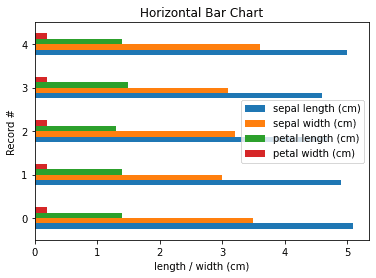NOTE

You can also use `df.plot.barh()` instead of `df.plot(kind='barh')` as they are equivalent. The same parameters can be used with both.

### Stacked

To create a stacked bar chart pass the parameter `stacked=True`:

``` ax = df[:5].plot(kind='bar', stacked=True, title='Stacked Bar Chart')ax.set_xlabel('Record #') # Add x-axis label ax.set_ylabel('length / width (cm)') # Add y-axis label ```

This produces the following: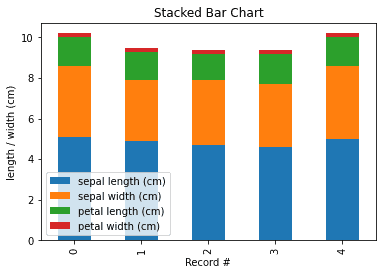## Box Plot

To create a box plot use `df.plot(kind='box')`:

``` ax = df.plot(kind='box', title='Box Plot')ax.set_xticklabels(['sepal length', 'sepal width', 'petal length', 'petal width']) # Specify xtick labelsax.set_ylabel('length / width (cm)') # Add y-axis label ```

This produces the following: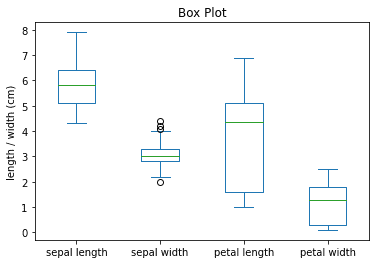We can see here that `petal length` shows high dispersion compared to the other features as indicated by the long box (representing the interquartile range). We also notice that `sepal width` seems to have a few outliers as represented by the circles which we may want to look into further.

NOTE

You can also use `df.plot.box()` instead of `df.plot(kind='box')` as they are equivalent. The same parameters can be used with both.

## Histogram

### Basic

To plot a histogram use `df.plot(kind='hist')`:

``` ax = df.plot(kind='hist', title='Histogram Plot')ax.set_xlabel('length / width (cm)') # Add x-axis label ax.set_ylabel('Frequency') # Add y-axis label ```

This produces the following: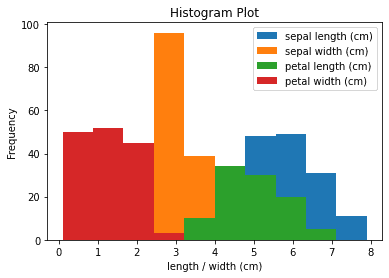Here we have plotted the frequency of four features on the same histogram. We can see the frequency of each interval for sepal length, sepal width, petal length, and petal width.

NOTE

You can also use `df.plot.hist()` instead of `df.plot(kind='hist')` as they are equivalent. The same parameters can be used with both.

### Transparent

To adjust the transparency, specify the `alpha` parameter:

``` ax = df.plot(kind='hist', title='Histogram Plot', alpha=0.4)ax.set_xlabel('length / width (cm)') # Add x-axis label ax.set_ylabel('Frequency') # Add y-axis label ```

This produces the following histogram with transparency 60% (i.e. 1 - alpha value):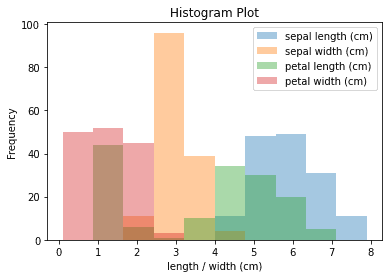Here adjusting the transparency is useful as we have overlapping bars due to the fact that we are plotting the distribution of four features on the same plot.

### Stacked

To create a stacked histogram pass the parameter `stacked=True`:

``` ax = df.plot(kind='hist', title='Stacked Histogram Plot', stacked=True)ax.set_xlabel('length / width (cm)') # Add x-axis label ax.set_ylabel('Frequency') # Add y-axis label ```

This produces the following: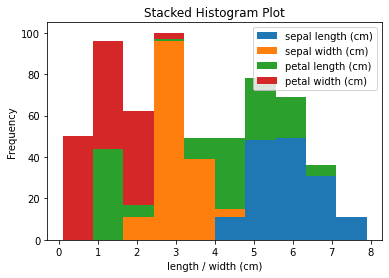## Kernel Density Estimation

To produce a Kernel Density Estimation plot:

``` ax = df.plot(kind='kde', title='Kernel Density Estimation')ax.set_xlabel('length / width (cm)') # Add x-axis label ax.set_ylabel('Frequency') # Add y-axis label ```

This produces the following: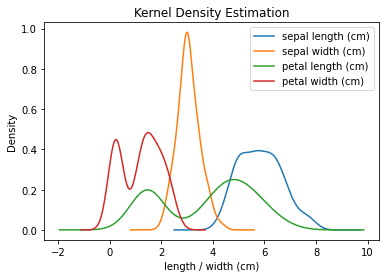NOTE

You can also use `df.plot.kde()` instead of `df.plot(kind='kde')` as they are equivalent. The same parameters can be used with both.

## Area Plot

To create an area plot:

``` ax = df.plot(kind='area', title='Area Plot')ax.set_xlabel('Record #') # Add x-axis label ax.set_ylabel('length / width (cm)') # Add y-axis label ```

This produces the following: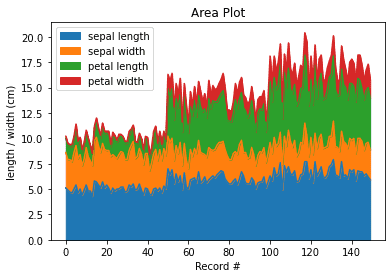By default a stacked area plot is produced, however, you can produce the unstacked equivalent by passing `stacked=False` to `df.plot(~)`.

## Scatter Plot

To create a scatter plot:

``` df.plot(kind='scatter', x='sepal length (cm)', y='petal length (cm)', title='Scatter Plot') ```

Here we use `'sepal length (cm)'` for the x-axis and `'petal length (cm)'` for the y-axis.

This produces the following: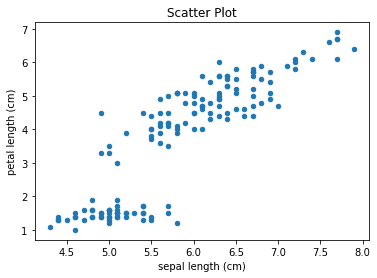## Pie Chart

Consider the following DataFrame:

``` import pandas as pddf = pd.DataFrame({'A':[1,2,3]}, index=['a','b','c'])df Aa 1b 2c 3 ```

### Values

To create a pie chart based on the values of elements in column `A`:

``` df.plot(kind='pie', subplots=True, title='Pie Chart') ```

This produces the following: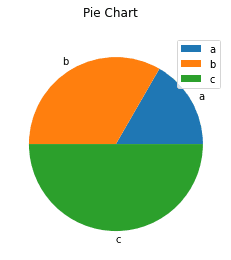NOTE

When creating a pie chart by using a DataFrame, you need to pass `subplots=True` to indicate that we should generate a pie chart for each column in the DataFrame. If you do not pass this, you will run into an error.

### Value counts

To create a pie chart based on the count of elements in column `A`:

``` import pandas as pddf = pd.DataFrame({'A':[1,2,3]}, index=['a','b','c'])df['A'].value_counts().plot(kind='pie', title='Pie Plot') ```

This produces the following: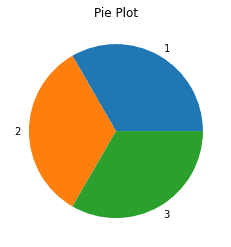Here we leverage the `value_counts(~)` method to count the occurrences of each element in column `A` of the DataFrame. In this example we have 1, 2 and 3 each occurring once resulting in an even breakdown in the pie chart.

### Percentage breakdown

To create a pie chart showing percentage breakdown of value counts in column `A`:

``` import pandas as pdimport matplotlib.pyplot as pltdf = pd.DataFrame({'A':[1,2,3]}, index=['a','b','c'])df['A'].value_counts().plot(kind='pie', title='Pie Plot', autopct='%1.1f%%') ```

This produces the following:The `autopct` parameter here allows us to specify a format string on how to display the percentage breakdowns of each category. In this example we display percentage values to 1 decimal place.

To remove the column name label appearing on the left side of the chart:

``` plt.ylabel("") ```

This produces the following: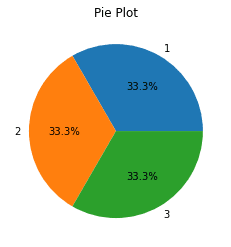Notice how the column name `A` that was appearing before on the left is no longer visible. What we are actually doing here to achieve this is setting the plot's y axis label as a blank string `""`.

# Useful Tips

For your convenience, recall we are working with the following DataFrame:

``` import numpy as npimport pandas as pddf = pd.read_csv('https://raw.githubusercontent.com/SkyTowner/sample_data/main/iris_dataset')df.head() sepal length (cm) sepal width (cm) petal length (cm) petal width (cm) target0 5.1 3.5 1.4 0.2 setosa1 4.9 3.0 1.4 0.2 setosa2 4.7 3.2 1.3 0.2 setosa3 4.6 3.1 1.5 0.2 setosa4 5.0 3.6 1.4 0.2 setosa ```

To adjust the plot size specify the `figsize` parameter when using the `df.plot(~)` method:

``` import matplotlib.pyplot as pltplt.figure()df.plot(figsize=(8,4), kind='scatter', x='sepal length (cm)', y='petal length (cm)', title='Scatter Plot')plt.show() ```

This outputs a graph with width 8 inches and height 4 inches.

To save the plot you have created use `plt.savefig(~)`:

``` import matplotlib.pyplot as pltplt.figure()df.plot(kind='scatter', x='sepal length (cm)', y='petal length (cm)', title='Scatter Plot')plt.savefig('my_scatterplot.png') ```

This will save a png file named "my_scatterplot.png" in the same directory as your Python script.

mail